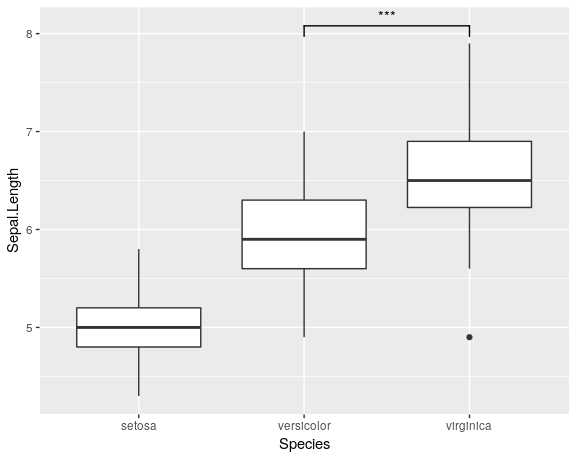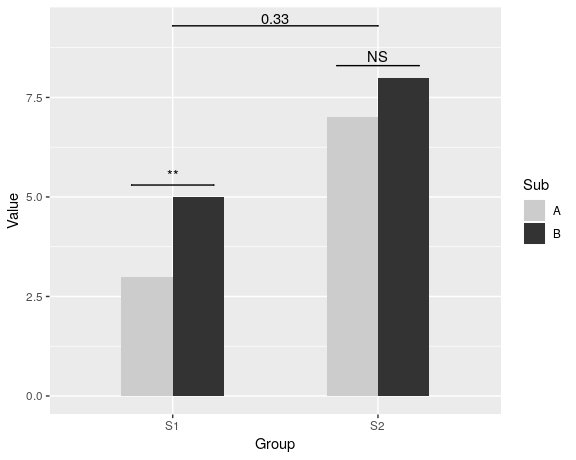ggsignif intro

2019-02-20

This package provides an extension for the popular ggplot2 package.

Often people are looking for a way to quickly annotate if the difference between 2 groups in a plot is significantly different (look at all those questions). The usual answer is along the lines of just manually adding lines and annotation to the plot.

But I believe that a fundamental strength of ggplot is that it allows one to quickly make advanced plots by combining layers of visualization, which can encapsulate complex statistical methods (geom_smooth, geom_contour etc.).

So I created this package which provides a single layer geom_signif which can calculate the significance of a difference between groups and add the annotation to the plot in a single line.

How to use it?

library(ggplot2)
library(ggsignif)

ggplot(iris, aes(x=Species, y=Sepal.Length)) +
geom_boxplot() +
geom_signif(comparisons = list(c("versicolor", "virginica")),
map_signif_level=TRUE)That’s it, it is really simple!

Sometimes one might need more advanced control over the display. To specify exactly where the bracket is drawn use the y_position, xmin and xmax parameters combined with a custom annotations. This is always necessary if geom_signif is combined with another layer that uses position="dodge", because it changes the location of the visual elements without updating the data.

dat <- data.frame(Group = c("S1", "S1", "S2", "S2"),
Sub   = c("A", "B", "A", "B"),
Value = c(3,5,7,8))

ggplot(dat, aes(Group, Value)) +
geom_bar(aes(fill = Sub), stat="identity", position="dodge", width=.5) +
geom_signif(y_position=c(5.3, 8.3), xmin=c(0.8, 1.8), xmax=c(1.2, 2.2),
annotation=c("**", "NS"), tip_length=0) +
geom_signif(comparisons=list(c("S1", "S2")),
y_position = 9.3, tip_length = 0, vjust=0.2) +
scale_fill_manual(values = c("grey80", "grey20"))For more detailed documentation of the available parameters see the man page for the geom_signif function (> ?geom_signif).